# RD Sharma Solutions for Class 6 Maths Chapter 15: Pair of Lines and Transversal Exercise 15.1

Exercise 15.1 has problems based on the concept of determining parallel lines in the given diagrams. The students are provided with a set of examples in order to make them familiar with the concepts. The two lines which do not intersect when extended in both directions are called parallel lines. The solutions are designed by faculty at BYJU’S based on the latest CBSE guidelines. Students can use RD Sharma Solutions Class 6 Maths Chapter 15 Pair of Lines and Transversal Exercise 15.1 PDF, which are provided here, to score well in their exam.

## RD Sharma Solutions for Class 6 Maths Chapter 15: Pair of Lines and Transversal Exercise 15.1 Download PDF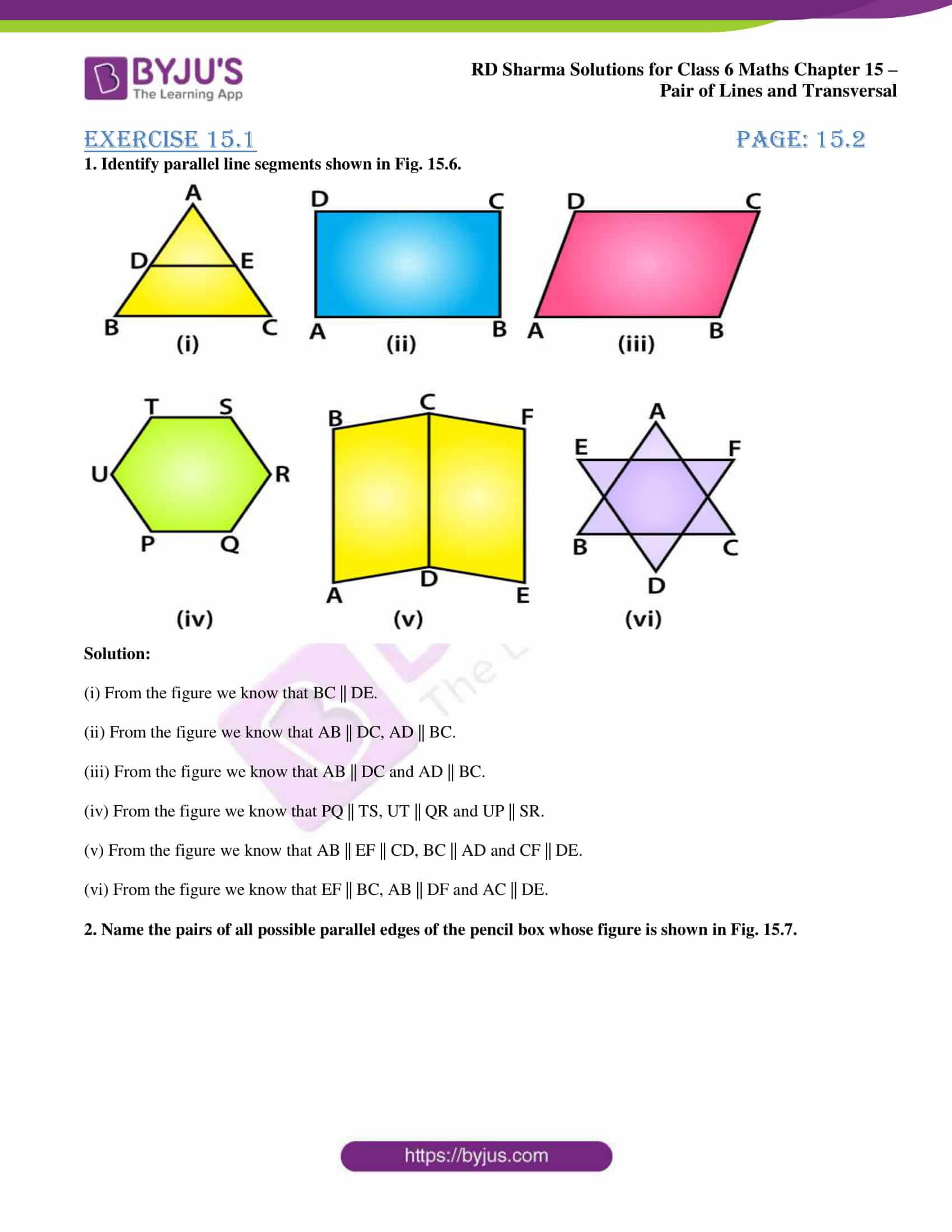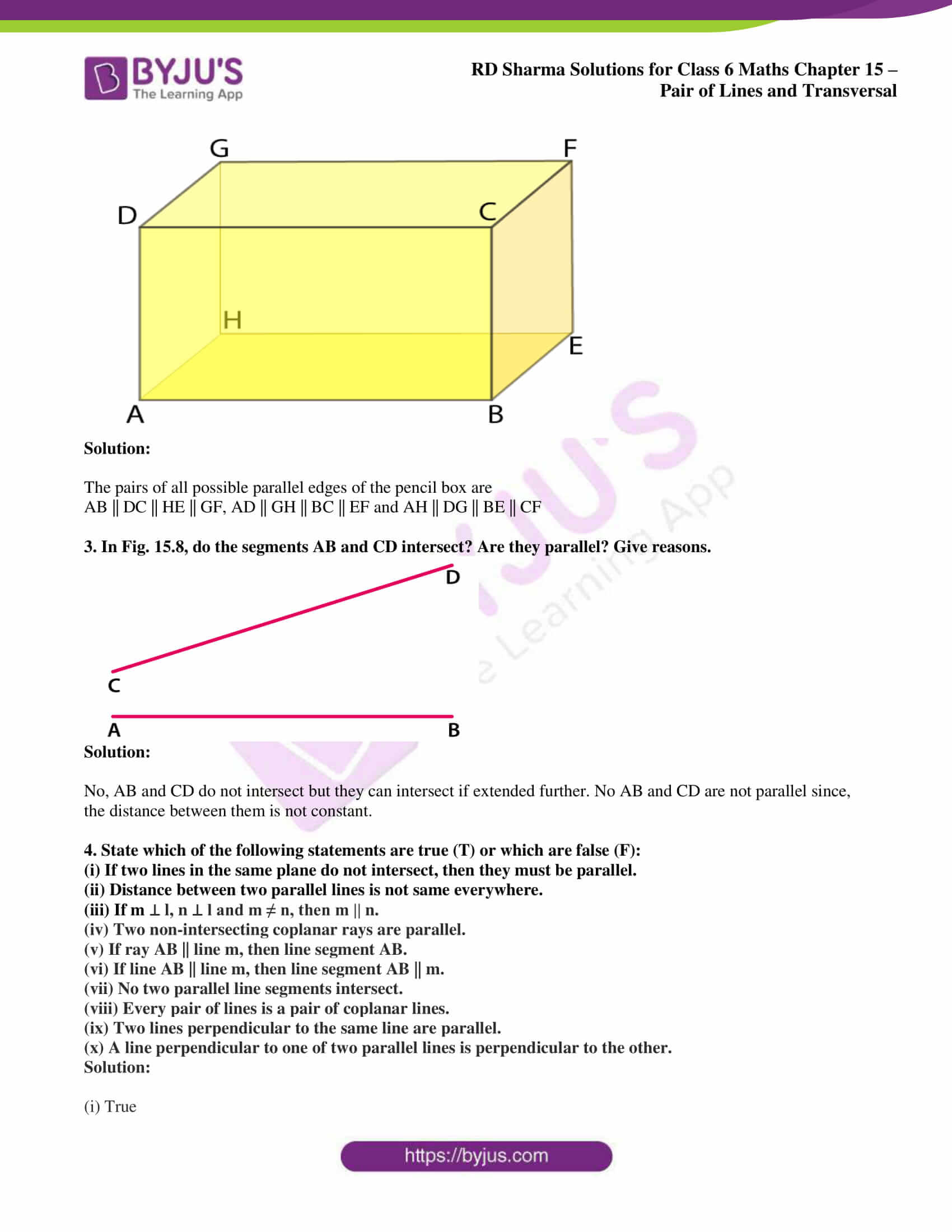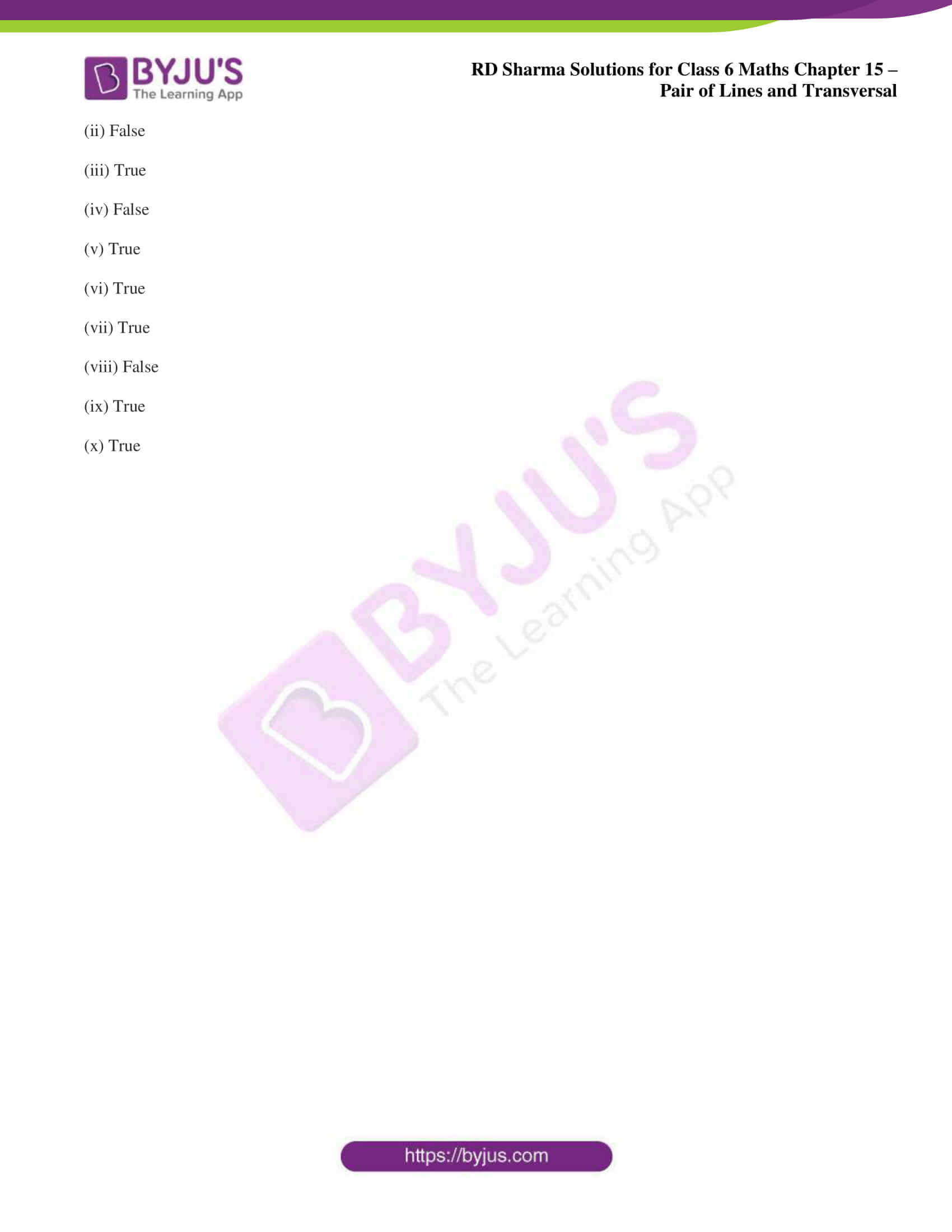### Exercise 15.1 page: 15.2

1. Identify parallel line segments shown in Fig. 15.6.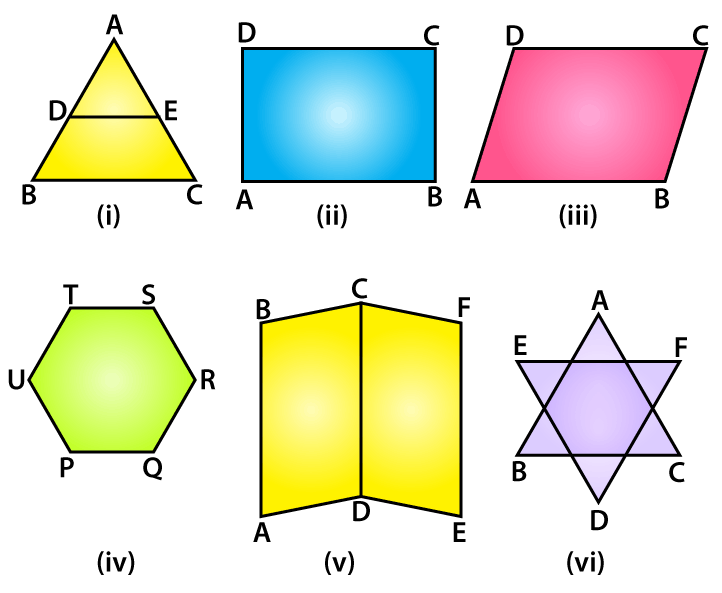Solution:

(i) From the figure we know that BC || DE.

(ii) From the figure we know that AB || DC, AD || BC.

(iii) From the figure we know that AB || DC and AD || BC.

(iv) From the figure we know that PQ || TS, UT || QR and UP || SR.

(v) From the figure we know that AB || EF || CD, BC || AD and CF || DE.

(vi) From the figure we know that EF || BC, AB || DF and AC || DE.

2. Name the pairs of all possible parallel edges of the pencil box whose figure is shown in Fig. 15.7.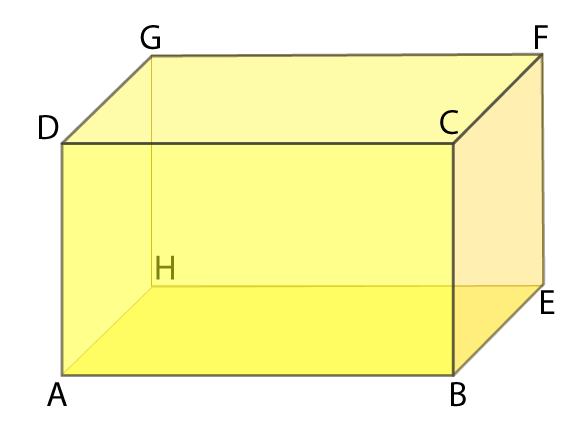Solution:

The pairs of all possible parallel edges of the pencil box are

AB || DC || HE || GF, AD || GH || BC || EF and AH || DG || BE || CF

3. In Fig. 15.8, do the segments AB and CD intersect? Are they parallel? Give reasons.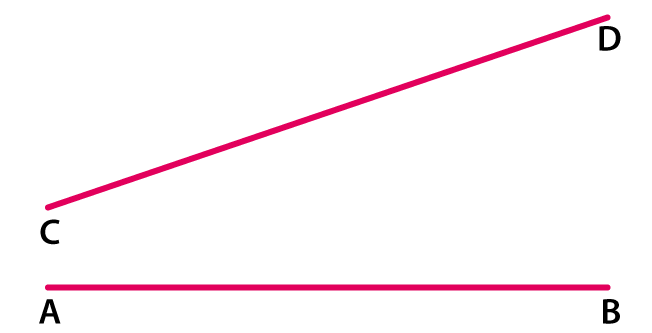Solution:

No, AB and CD do not intersect but they can intersect if extended further. No AB and CD are not parallel since, the distance between them is not constant.

4. State which of the following statements are true (T) or which are false (F):

(i) If two lines in the same plane do not intersect, then they must be parallel.

(ii) Distance between two parallel lines is not same everywhere.

(iii) If m ⊥ l, n ⊥ l and m ≠ n, then m || n.

(iv) Two non-intersecting coplanar rays are parallel.

(v) If ray AB || line m, then line segment AB.

(vi) If line AB || line m, then line segment AB || m.

(vii) No two parallel line segments intersect.

(viii) Every pair of lines is a pair of coplanar lines.

(ix) Two lines perpendicular to the same line are parallel.

(x) A line perpendicular to one of two parallel lines is perpendicular to the other.

Solution:

(i) True

(ii) False

(iii) True

(iv) False

(v) True

(vi) True

(vii) True

(viii) False

(ix) True

(x) True